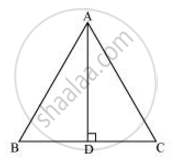# ABC is an equilateral triangle of side 2a. Find each of its altitudes. - Mathematics

ABC is an equilateral triangle of side 2a. Find each of its altitudes.

#### SolutionLet AD be the altitude in the given equilateral triangle, ΔABC.

We know that altitude bisects the opposite side.

∴ BD = DC = a

Applying pythagoras theorem we obtain

⇒ AD2 + a2 = (2a)2

⇒ AD2 + a2 = 4a2

⇒ AD =asqrt3

In an equilateral triangle, all the altitudes are equal in length. Therefore, the length of each altitude will be sqrt3a

Concept: Right-angled Triangles and Pythagoras Property
Is there an error in this question or solution?

#### APPEARS IN

NCERT Class 10 Maths
Chapter 6 Triangles
Exercise 6.5 | Q 6 | Page 150